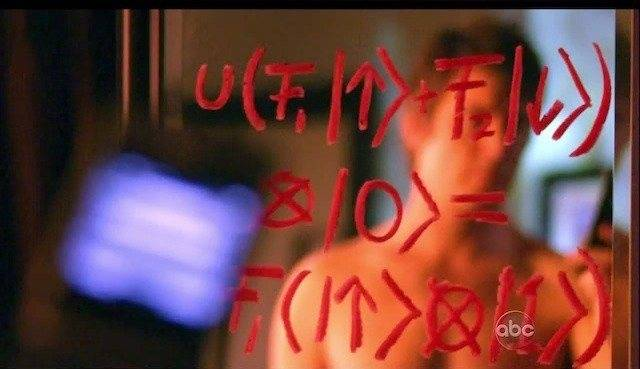# Discover the Equation: Solving the Mystery of This Math Problem

• CosmicVoyager

#### CosmicVoyager

Greetings,

Would anyone happen to have any clue as to what equation or what sort of math this is?

Thanks#### Attachments

Last edited:
Attachment didn't work for me.

Looks like basic quantum information, entanglement of states and such

$\otimes$ is a tensor product
$|1\rangle$ is a quantum state

## 1. What is "Discover the Equation: Solving the Mystery of This Math Problem" about?

"Discover the Equation: Solving the Mystery of This Math Problem" is a book that explores the history and significance of a particular mathematical equation or problem. It delves into the process of solving the problem and the impact it has had on the field of mathematics.

## 2. Who is the author of "Discover the Equation: Solving the Mystery of This Math Problem"?

The author of "Discover the Equation: Solving the Mystery of This Math Problem" is a renowned mathematician or scientist who has expertise in the specific problem being discussed in the book. The author's name is usually listed on the cover or in the book's introduction.

## 3. What makes "Discover the Equation: Solving the Mystery of This Math Problem" different from other math books?

"Discover the Equation: Solving the Mystery of This Math Problem" is different from other math books because it focuses on a specific problem or equation rather than covering a broad range of mathematical concepts. It also delves into the history and significance of the problem, providing a more in-depth understanding of its impact.

## 4. Is "Discover the Equation: Solving the Mystery of This Math Problem" suitable for non-mathematicians?

Yes, "Discover the Equation: Solving the Mystery of This Math Problem" can be enjoyed by non-mathematicians as well. While some knowledge of mathematical concepts may be helpful, the book is written in a way that is accessible to a general audience. The author often explains complex ideas in a clear and understandable manner.

## 5. Can "Discover the Equation: Solving the Mystery of This Math Problem" help with improving math skills?

"Discover the Equation: Solving the Mystery of This Math Problem" is not a textbook or a guide to improving math skills. However, it can provide insight into problem-solving strategies and give a deeper understanding of mathematical concepts. It may also inspire readers to explore more mathematical problems and improve their skills through practice and study.